# One-map two-clock relativity for introductory physics classes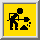## Abstract

This paper presents some ideas which might assist educators incorporating special relativity into an introductory physics course. One can define the ``proper'' time and velocity, as well as the ``coordinate'' time and velocity, of a traveler using only distances measured with respect to a single ``map'' frame. When this is done, the relativistic equations for momentum, energy, constant acceleration, and force take take on forms strikingly similar to their Newtonian counterparts. Thus high-school and college students not ready for Lorentz transforms may solve relativistic versions of many single-frame Newtonian problems they have mastered. We further show that multi-frame calculations (like the velocity-addition rule) acquire simplicity and/or utility not found using coordinate-velocity alone. From physics/9611011 (xxx.lanl, NM, 1996).

PACS: 03.30.+p, 01.40.Gm, 01.55.+b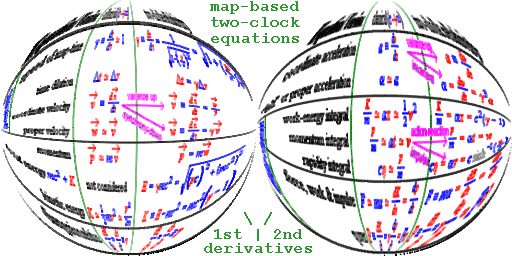• I. Introduction

• II. A traveler, one map, and two clocks
• A. Developing the basic equations
• B. Basic classroom applications

• III. Acceleration and force with one map and two clocks
• A. Developing the acceleration equations
• B. Classroom application of relativistic acceleration and force

• IV. Problems involving more than one map
• A. Development of multi-map equations
• B. Classroom applications involving more than one map-frame

• V. Conclusions
• VI. Acknowledgements
• VII. Appendix A: The 4-vector perspective
• VIII. References
• IX. Tables

• by Phil Fraundorf, Dept. of Physics & Astronomy, University of Missouri-StL
• Citable as arXiv:physics/9611011 (xxx.lanl.gov archive, Los Alamos, NM, 1996).
• Related papers: modernizing Newton, anyspeed modeling, and anticipation.
• A copy optimized for printing with Adobe's free Acrobat PDF reader is here.
• Here are many links on anyspeed motion from a single frame's vantage point.
• You might also enjoy some of our nanoexploration and information physics links.
• Symbols: This paper has a notation shift from earlier papers. Here coordinate {time, velocity}={t, v}, and proper {time, velocity}={tau, w}. Galilean-kinematic {time,velocity} are not used.
• Version release date: 16 Apr 2005.
• At UM-StLouis see also: a1toc, cme, i-fzx, progs, stei-lab, & wuzzlers.
• For URL source, cite http://www.umsl.edu/~fraundor/twoclock.html
• Mindquilts site page requests to UM-StL ~2000/day approaching a million per year.
• Requests for a "stat-counter linked subset of pages" since 4/7/2005: .

## I. Introduction

Since the 1920's, it has been known that classical Newtonian laws depart from a description of reality, as velocity approaches the speed of light. This divergence between Newtonian and relativistic physics was one of the most remarkable discoveries of this century. Yet, more than 70 years later, most American high schools and colleges teach introductory students Newtonian dynamics, but then fail to teach students how to solve those same problems relativistically. When relativity is discussed at all, it often entails only a rather abstract introduction to Lorentz transforms, relegated to the back of a chapter (or the back of a book).

This is in part due to the facts that: (i) Newton's laws work so well in routine application, and (ii) introductions to special relativity (often patterned after Einstein's introductions to the subject) focus on discovery-philosophy rather than applications. Caution, and inertia associated with old habits, may play a role as well. It is nonetheless unfortunate because teaching Newtonian solutions without relativistic ones at best leaves the student's education out-of-date, and deprives them of experiences which might spark an interest in further physics education. At worst, partial treatments may replace what is missing with misconceptions about the complexity, irrelevance, and/or the limitations of relativity in the study, for example, of simple things like uniform acceleration.

Efforts to link relativistic concepts to classical ones have been with us from the beginning. For example, the observation that relativistic objects behave at high speed as though their inertial mass increases in the p = mv expression, led to the definition (used in many early textbooks {e.g. French}) of relativistic mass m' = m gamma. Such efforts are worthwhile because they can: (A) potentially allow the introduction of relativity concepts at an earlier stage in the education process by building upon already-mastered classical relationships, and (B) find what is fundamentally true in both classical and relativistic approaches. The concepts of transverse (m' ) and longitudinal (m'' = m gamma^3) masses have similarly been used {e.g. Blatt} to preserve relations of the form Fx=max for forces perpendicular and parallel, respectively, to the velocity direction.

Unfortunately for these relativistic masses, no deeper sense has emerged in which the mass of a traveling object either changes, or has directional dependence. Such masses allow familiar relationships to be used in keeping track of non-classical behaviors (item A above), but do not (item B above) provide frame-invariant insights or make other relationships simpler as well. Hence majority acceptance of their use seems further away now {e.g. Adler} than it did several decades ago {Goldstein}.

A more subtle trend in the literature has been toward the definition of a quantity called proper velocity {cf. SearsBrehme, Shurcliff}, which can be written as w = gamma v. We use the symbol w here because it is not in common use elsewhere in relativity texts, and because w resembles gamma v from a distance. This quantity also allows the momentum expression above to be written in classical form as a mass times a velocity, i.e. as p = mw. Hence it serves one of the ``item A'' goals served by m' above. However, it remains an interesting but ``homeless'' quantity in the present literature. In other words, proper-velocity differs fundamentally from the familiar coordinate-velocity, and unlike the latter has not in textbooks been linked to a particular reference frame. After all, it uses distance measured in an inertial frame but time measured on the clocks of a moving and possibly accelerated observer. However, there is also something deeply physical about proper-velocity. Unlike coordinate-velocity, it is a synchrony-free (i.e. local-clock only) {cf. Winnie, Ungar1} means of quantifying motion. Moreover, A. Ungar has recently made the case {Ungar2} that proper velocities, and not coordinate velocities, make up the gyrogroup analog to the velocity group in classical physics. If so, then, is it possible that introductory students might gain deeper physical insight via its use?

The answer is yes. We show here that proper-velocity, when introduced as part of a ``one-map two-clock'' set of time/velocity variables, allows us to introduce relativistic momentum, time-dilation, and frame-invariant relativistic acceleration/force into the classroom without invoking discussion of multiple inertial frames or the abstract mathematics of Lorentz transformation (item A above). Moreover, through use of proper-velocity many relationships (including those like velocity-addition, which require multiple frames or more than one ``map'') are made simpler and sometimes more useful. When one simplification brings with it many others, this suggests that ``item B'' insights may be involved as well. The three sections to follow deal with the basic, acceleration-related, and multi-map applications of this ``two-clock'' approach by first developing the equations, and then discussing classroom applications.

## V. Conclusions

We show in this paper that a one-map two-clock approach, using both proper and coordinate velocities, lets students tackle time dilation as well as momentum and energy conservation problems without having to first master concepts which arise when considering more than one inertial frame (like Lorentz transforms, length contraction, and frame-dependent simultaneity). The cardinal rule to follow when doing this is simple: All distances must be defined with respect to a ``map'' drawn from the vantage point of a single inertial reference frame.

We show further that a frame-invariant proper acceleration 3-vector has three simple integrals of the motion in terms of these variables. Hence students can speak of the proper acceleration and force 3-vectors for an object in map-independent terms, and solve relativistic constant acceleration problems much as they now do for non-relativistic problems in introductory courses.

We have provided some examples of the use of these equations for high school and college introductory physics classes, as well as summaries of equations for the simple unidirectional motion case (Tables I, II, and III). In the process, one can see that the approach does more than ``superficially preserve classical forms''. Not just one, but many, classical expressions take on relativistic form with only minor change. In addition, interesting physics is accessible to students more quickly with the equations that result. The relativistic addition rule for proper velocities is a special case of the latter in point. Hence we argue that the trend in the pedagogical literature, away from relativistic masses and toward use of proper time and velocity in combination, may be a robust one which provides: (B) deeper insight, as well as (A) more value from lessons first-taught.

## VI. Acknowledgments

My thanks for input relevant to this paper from G. Keefe, W. A. Shurcliff, E. F. Taylor, and A. A. Ungar. The work has benefited indirectly from support by the U.S. Department of Energy, the Missouri Research Board, as well as Monsanto and MEMC Electronic Materials Companies. It has benefited most, however, from the interest and support of students at UM-St. Louis.

## VIII. References

• A. P. French, Special Relativity (W. W. Norton, NY, 1968), p. 22.
• F. J. Blatt, Modern Physics (McGraw-Hill, NY, 1992).
• C. G. Adler, ``Does mass really depend on velocity, dad?'', Amer. J. Physics, 55 (1987) 739-743.
• H. Goldstein, Classical Mechanics, 7th printing (Addison-Wesley, Reading MA, 1965), p. 205.
• Sears and Brehme, Introduction to the Theory of Relativity (Addison Wesley, 1968).
• W. A. Shurcliff, Special Relativity: The Central Ideas (19 Appleton St., Cambridge MA 02138, 1996).
• J. A. Winnie, ``Special relativity without one-way velocity assumptions, Part I and II'', Philos. Sci., 37 (1970) 81-99 and 223-228.
• A. A. Ungar, ``Formalism to deal with Reichenbach's special theory of relativity'', Found. Phys., 21 (1991) 691-726.
• A. A. Ungar, ``Gyrogroup axioms for the abstract Thomas precession and their use in relativistic physics and hyperbolic geometry'', Found. Phys., submitted (1996).
• P. Fraundorf, "Non-coordinate time/velocity pairs in special relativity", General Relativity & Quantum Cosmology gr-qc/9607038, (xxx.lanl.gov archive, Los Alamos NM, 1996).
• F. Taylor and J. A. Wheeler, Spacetime Physics (W. H. Freeman, San Francisco, 1963 and 1992).
• C. Lagoute and E. Davoust, ``The interstellar traveler'', Am. J. Phys., 63 (1995) 221.

## IX. Tables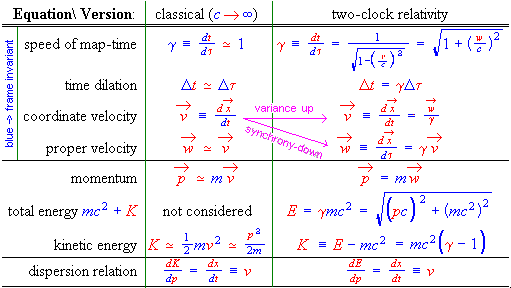Table I. Equations involving velocities, times, momentum and energy, in classical and two-clock relativistic form.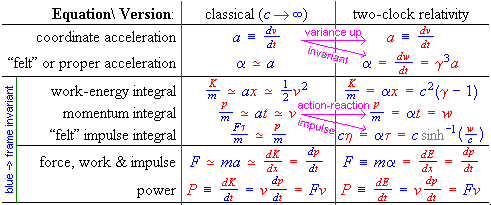Table II. Unidirectional motion equations involving constant acceleration from rest, and ``2nd law'' dynamics, in classical and two-clock relativistic form. The full (3+1)D version, with equalities valid for any map-frame, differs in that proper force & acceleration are divided by gammaperp relative to momentum & velocity, but multiplied by gammaperp relative to energy & dv/dt.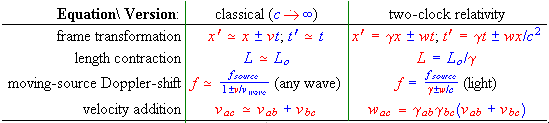Table III. Unidirectional motion equations involving distances measured in more than one map-frame, in classical and two-clock relativistic form.

For more, see our web construction table of contents on this subject. Please share your thoughts using our review template, or send comments, answers to problems posed, and/or complaints, to philf@newton.umsl.edu. This page contains original material, so if you choose to echo in your work, in print, or on the web, a citation would be cool. ` (Thanks. /philf :)`